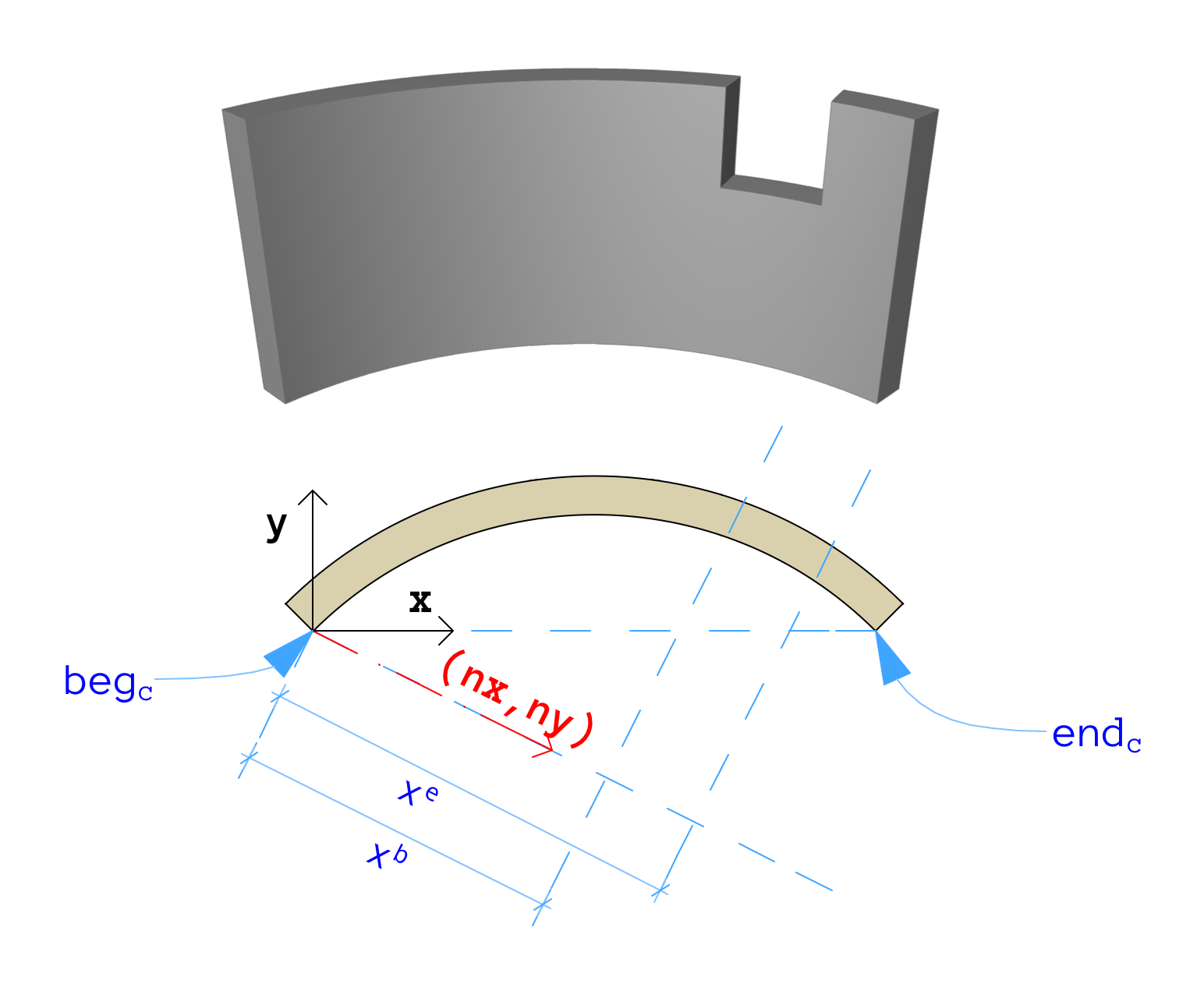## API_​Gable

Defines a cutting plane applied to spatial elements.

```    typedef struct {
double             a;
double             b;
double             c;
double             d;
double             xb;
double             xe;
double             nx;
double             ny;
short              cutElem;
short              filler_1;
API_AttributeIndex material;
API_Coord          **coords;
Int32              **pends;
API_PolyArc        **parcs;
} API_Gable;
```

#### Members

a, b, c, d
Plane coefficients relative to the parent element.
xb, xe
Distance of vertical cut planes from local origin.
nx, ny
Normal vector of vertical cut planes (nz always 0.0).
cutElem
Element the gable is generated from (output only).
material
Material for the cut surface (output only).
coords
Coordinate array, not nullptr if cut is limited to a polygon.
pends
parcs

#### Remarks

The a, b, c, d plane coefficients are the parameters of the plane equation:

ax + by + cz = d

Where x, y and z are points in 3D space, a, b and c are the x, y and z components of the surface normal, and d is the distance value. A point whose components are put into x, y and z is on the plane if the above equality is satisfied.

All gable data is defined in the local coordinate system of the element.

The picture below shows how gable parameters should be interpreted for curved walls:Note that the coordinates of the limit polygon are transformed (shifted and rotated) into a position relative to the first edge of the zone polygon. To get the real gable polygon coordinates you should transform them back.

Check the Dump Zone function in the Element_Manager example project.

#### Requirements

Version: API 2.1 or later